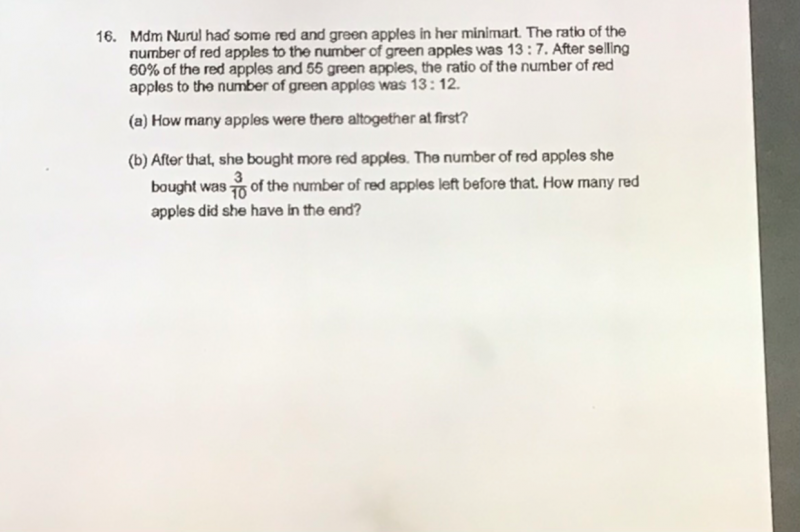# QuestionRed  Apples                   Green Apples

Before            13u                                        7u

Then      -0.6 x 13=7.8u                           -55

After                13p                                      12p

13u-7.8u=5.2u =13p => p = (5.2/13) u = 0.4u

7u-55 = 12p

7u-55 = 12(0.4)u = 4.8u

7u-4.8u = 2.2u = 55 => u = 25

(a) Red apples at first = 13u = 13 x 25 = 325

(b) Reminder Red apples = 5.2 x 25 = 130

(3/10 ) x 130 = 2 x 13 = 39 new Red apples added

Hence, in the end the number of Red apples she had = 130 + 39 = 169

0 Replies 1 Like